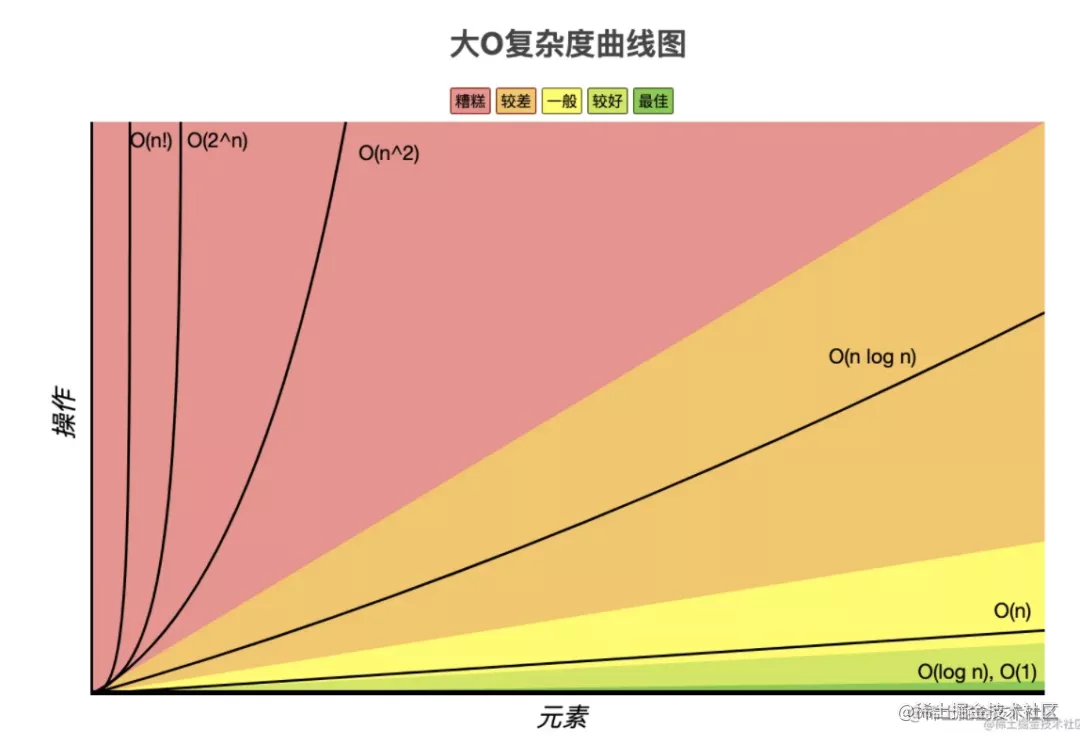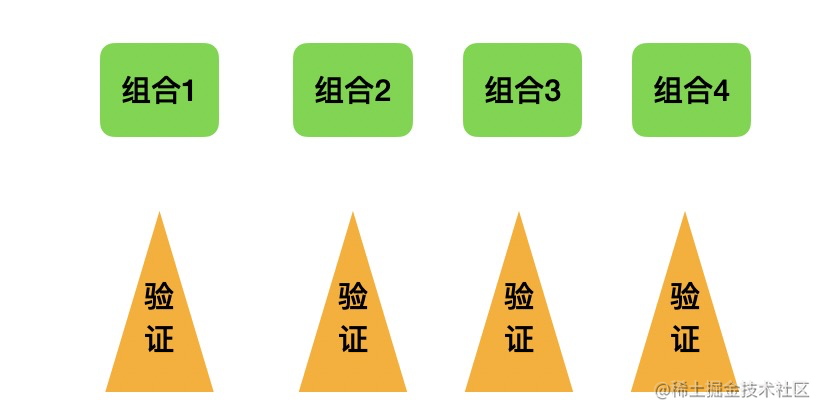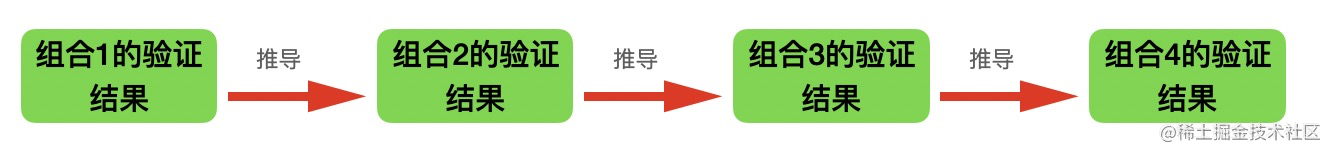# 枚举之后再验证性能太差？来试下动态规划

• 一个字符串可以有 n 种子串，让我们从中选出最长的回文串。（回文串是指 abccba 这种反过来和原字符串相等的字符串）

• n 个物品之间有很多种组合方案，让我们选出满足满足重量小于某个值的最大价值的组合方案``````if (str[i] === str[j] && isHuiwen[i+1][j-1]) {
isHuiwen[i][j] = true;
}`````` if (j < w[i]) {
maxValue[i][j] = maxValue[i -1][j]
}

`````` if (j >= w[i]) {
maxValue[i][j] = Math.max(maxValue[i][j - w[i]] + val[i], maxValue[i-1][j])
}

`````` if (j < w[i]) {
maxValue[i][j] = maxValue[i -1][j]
} else {
maxValue[i][j] = Math.max(maxValue[i][j - w[i]] + val[i], maxValue[i-1][j])
}